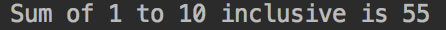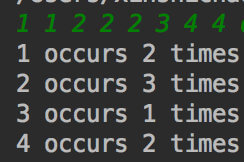# 【C++基础】第三课：循环语句与判断语句

## while语句，for语句，if语句

Posted by x-jeff on March 10, 2019

【C++基础】系列博客为参考《C++ Primer中文版（第5版）》C++11标准）一书，自己所做的读书笔记。

# 1.循环语句

## 1.1.while语句

while语句反复执行一段代码，直至给定条件为假为止。

1
2
3
4
5
6
7
8
9
10
11
12
#include <iostream>
int main()
{
int sum=0,val=1;
while (val<=10)
{
sum+=val;
++val;
}
std::cout<<"Sum of 1 to 10 inclusive is "<<sum<<std::endl;
return 0;
}👉while语句的形式为：

1
2
while (condition)
statement


🚩语句块(block)：用花括号包围起来的零条或多条语句的序列。语句块也是语句的一种，在任何要求使用语句的地方都可以使用语句块。

🚩复合赋值运算符+=sum+=val相当于sum=sum+val

🚩++--

• 前缀递增运算符++val先对val执行+1操作，然后再返回val的值。
• 后缀递增运算符val++先返回val的值，然后再对val执行+1操作。
• 前缀递减运算符--val先对val执行-1操作，然后再返回val的值。
• 后缀递减运算符val--先返回val的值，然后再对val执行-1操作。

1. i=++j;得到$i=2,j=2$
2. i=j++;得到$i=1,j=2$
3. i=--j;得到$i=0,j=0$
4. i=j--;得到$i=1,j=0$

## 1.2.for语句

1
2
3
4
5
6
7
8
9
#include<iostream>
int main()
{
int sum=0;
for(int val=1;val<=10;++val)
sum+=val;
std::cout<<"Sum of 1 to 10 inclusive is "<<sum<<std::endl;
return 0;
}


• 循环头(由三部分组成)
• 一个初始化语句(init-statement)：初始化语句只在for循环入口处执行一次。
• 一个循环条件(condition)：循环体每次执行前都会先检查循环条件。只要满足循环条件，就会执行for循环体。
• 一个表达式(expression)：表达式在for循环体之后执行。执行完表达式后，for语句重新检测循环条件。
• 循环体

## 1.3.实战应用：读取数量不定的输入数据

1
2
3
4
5
6
7
8
9
#include <iostream>
int main()
{
int sum=0,value=0;
while(std::cin>>value)
sum+=value;
std::cout<<"Sum is: "<<sum<<std::endl;
return 0;
}


## 1.4.关于编译器

1. 语法错误(syntax error)
2. 类型错误(type error)
3. 声明错误(declaration error)

“编辑-编译-调试”(edit-compile-debug)周期。

# 2.判断语句

1
2
3
4
5
6
7
8
9
10
11
12
13
14
15
16
17
18
19
#include <iostream>
int main()
{
int currVal=0,val=0;
if(std::cin>>currVal){
int cnt=1;
while(std::cin>>val){
if(val==currVal)
++cnt;
else{
std::cout<<currVal<<" occurs "<<cnt<<" times"<<std::endl;
currVal=val;
cnt=1;
}
}
std::cout<<currVal<<" occurs "<<cnt<<" times"<<std::endl;
}
return 0;
}⚠️区分===：C++用=进行赋值，用==作为相等运算符。